# Model (in logic)

An interpretation of a formal language satisfying certain axioms (cf. Axiom). The basic formal language is the first-order languageof a given signatureincluding predicate symbols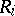,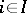, function symbols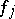,, and constants,. A model of the language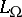is an algebraic system of signature.

Let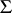be a set of closed formulas in. A model foris a model forin which all formulas fromare true. A setis called consistent if it has at least one model. The class of all models ofis denoted by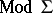. Consistency of a setmeans that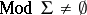.

A class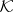of models of a languageis called axiomatizable if there is a setof closed formulas ofsuch that. The setof all closed formulas ofthat are true in each model of a given classof models ofis called the elementary theory of. Thus, a classof models ofis axiomatizable if and only if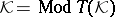. If a classconsists of models isomorphic to a given model, then its elementary theory is called the elementary theory of this model.

Let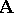be a model ofhaving universe. One may associate to each element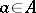a constantand consider the first-order language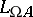of signaturewhich is obtained fromby adding the constants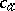,.is called the diagram language of the model. The set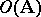of all closed formulas ofwhich are true inon replacing each constantby the corresponding elementis called the description (or elementary diagram) of. The setof those formulas fromwhich are atomic or negations of atomic formulas is called the diagram of.

Along with models of first-order languages, models of other types (infinitary logic, intuitionistic logic, many-sorted logic, second-order logic, many-valued logic, and modal logic) have also been considered.

For references see Model theory.